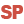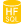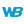Home |Sign in | EnglishHelpWLanguageDatabase managementManagement of statisticsHStatCalculatePresentationExampleSee alsoStatCalculateStatDateStatNbDuplicatesStatNbRecStatNbRecRangeStatTimeHStatCalculateHStatDateHStatNbDuplicatesHStatNbRecHStatNbRecRangeHStatTime
 WINDEVWEBDEVWINDEV MobileOthersHStatCalculate (Function) In french: HStatCalculeAvailable only with these kinds of connection Performs various statistical calculations on the file keys.Versions 16 and laterThis function is equivalent to HAccelerateSpeed.New in version 16This function is equivalent to HAccelerateSpeed.This function is equivalent to HAccelerateSpeed.These statistics are used when implementing a filter, creating a query or creating a view on the data file. The HFSQL engine analyzes the selection condition, then uses these statistics to define the most discriminating items that will be used to optimize the browse operations on data files.The statistics are used to get information about the content of the data file when they are calculated. Therefore, the more modifications are performed in the file after this calculation, the less these statistics represent the true content of the data file. The more records the data file contains, the less the modification of a single record impacts the overall statistics.Caution: HStatCalculate locks the data file in write mode while the statistics are calculated (a file browse is performed for each file key).The statistics can be calculated in background task without locking the data file in write mode. Remark: In order for the statistical calculation to be efficient, this calculation must be performed on all data files (for a multi-file query for example).Remark: From version 19, HFSQL is the new name of HyperFileSQL.Versions 21 and laterThis function is now available to perform statistical calculations on the data files of an HFSQL cluster.New in version 21This function is now available to perform statistical calculations on the data files of an HFSQL cluster.This function is now available to perform statistical calculations on the data files of an HFSQL cluster.Example // Statistics on the CustName key of the Customer data fileHStatCalculate(Customer, CustName) Syntax = HStatCalculate([ [,  [,